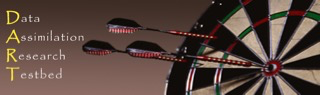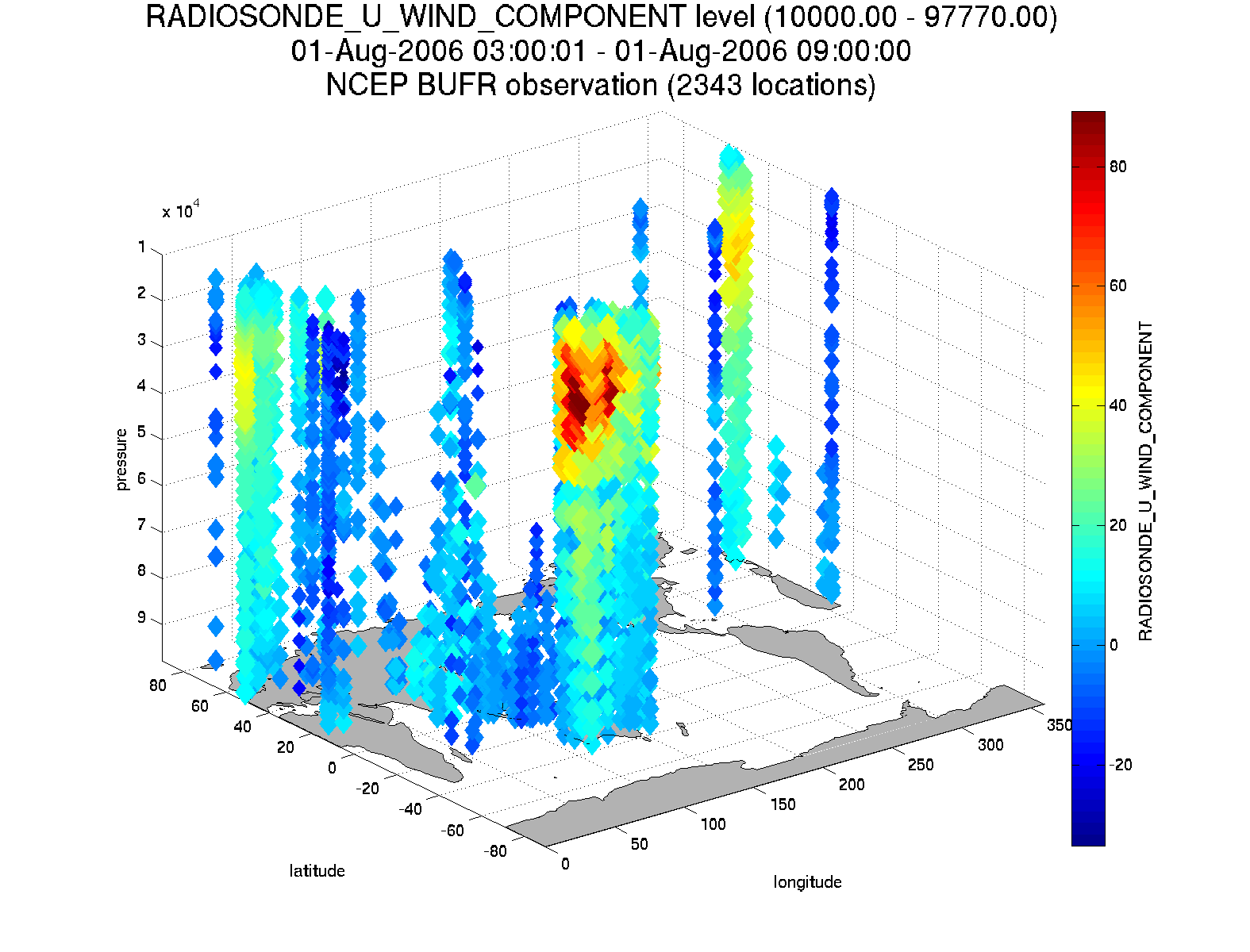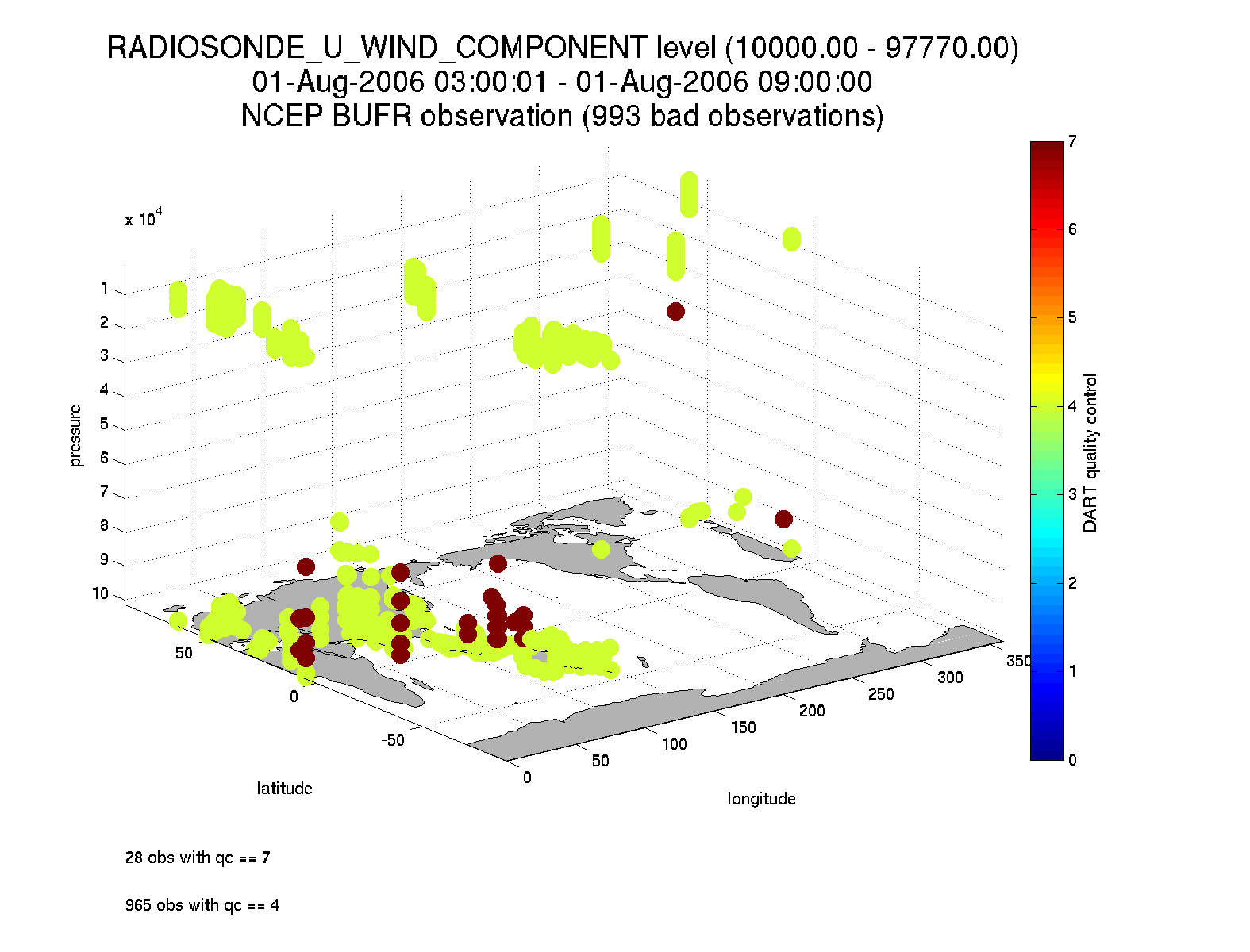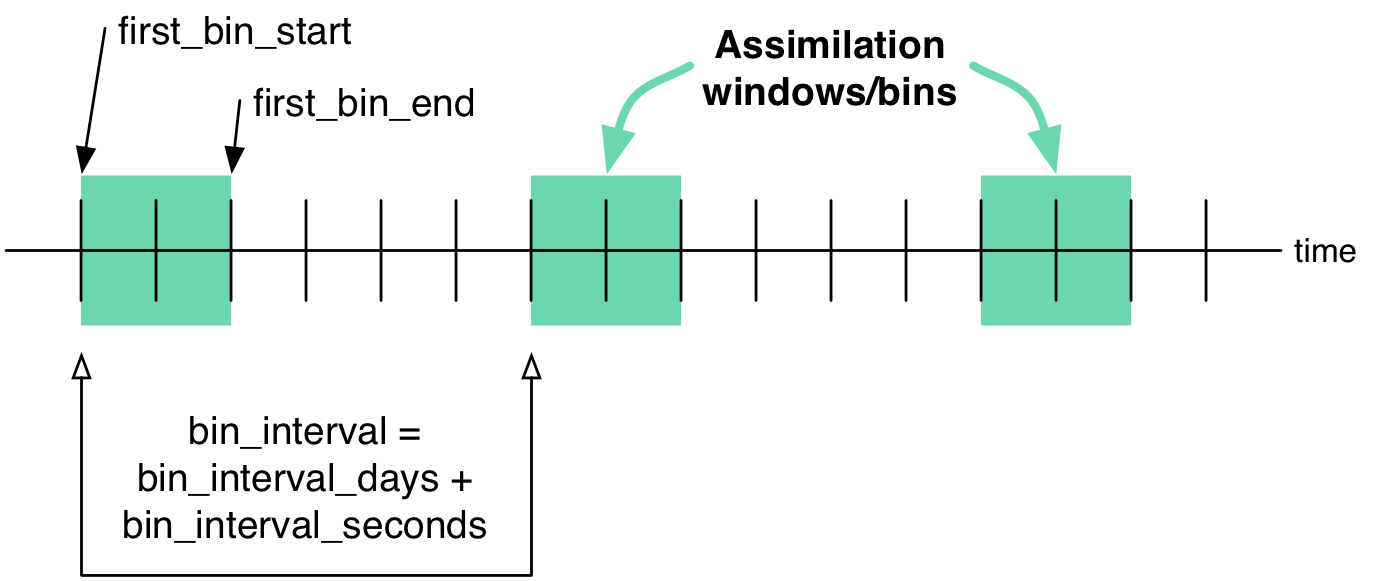# PROGRAM obs_seq_to_netcdfJump to DART Documentation Main Index
NAMELIST / MODULES / FILES / USAGE / REFERENCES / ERRORS / PLANS / PRIVATE COMPONENTS / TERMS OF USE

## Overview

obs_seq_to_netcdf is a routine to extract the observation components from observation sequence files and write out netCDF files that can be easily digested by other applications. This routine will allow you to plot the spatial distribution of the observations and be able to discern which observations were assimilated or rejected, for example. Here are some graphics from DART/diagnostics/matlab/plot_obs_netcdf.m.The intent is that user input is queried and a series of output files - one per assimilation cycle - will contain the observations for that cycle. It is hoped this will be useful for experiment design or, perhaps, debugging. This routine is also the first to use the new schedule_mod module which will ultimately control the temporal aspects of the assimilations (i.e. the assimilation schedule).

There is also a facility for exploring the spatial distributions of quantities like bias between the ensemble mean and the observations: DART/diagnostics/matlab/plot_obs_netcdf_diffs.m.

Required namelist interfaces &obs_seq_to_netcdf and &schedule_nml are read from file input.nml.

### What's on the horizon ...

obs_seq_to_netcdf is a step toward encoding our observations in netCDF files.

The dependence on the threed_sphere/location_mod.f90 has been removed. This program will work with any location_mod.f90. Also, this program no longer tries to construct 'wind' observations from horizontal components since the program really should be faithful to preserving exactly what is in the input file. i.e. We're not making stuff up.

There are several Matlab scripts that understand how to read and plot observation data in netcdf format. See the link_obs.m script that creates several linked figures with the ability to 'brush' data in one view and have those selected data (and attributes) get highlighted in the other views.

[top]

## NAMELIST

This namelist is read from the file input.nml. Namelists start with an ampersand '&' and terminate with a slash '/'. Character strings that contain a '/' must be enclosed in quotes to prevent them from prematurely terminating the namelist.

```&obs_seq_to_netcdf_nml
obs_sequence_name = 'obs_seq.final',
obs_sequence_list = '',
append_to_netcdf  = .false.,
lonlim1    =    0.0,
lonlim2    =  360.0,
latlim1    =  -90.0,
latlim2    =   90.0,
verbose    = .false.
/
```

The allowable ranges for the region boundaries are: latitude [-90.,90], longitude [0.,360.] ... but it is possible to specify a region that spans the dateline by specifying the lonlim2 to be less than lonlim1.

You can only specify either obs_sequence_name or obs_sequence_list -- not both.

Item Type Description
obs_sequence_name character(len=256) Name of an observation sequence file(s). This may be a relative or absolute filename. If the filename contains a '/', the filename is considered to be comprised of everything to the right, and a directory structure to the left. The directory structure is then queried to see if it can be incremented to handle a sequence of observation files. The default behavior of obs_seq_to_netcdf is to look for additional files to include until the files are exhausted or an obs_seq.final file is found that contains observations beyond the timeframe of interest.
e.g. 'obsdir_001/obs_seq.final' will cause obs_seq_to_netcdf to look for 'obsdir_002/obs_seq.final', and so on.
If this is specified, 'obs_sequence_list' must be set to ' '.
obs_sequence_list character(len=256) Name of an ascii text file which contains a list of one or more observation sequence files, one per line. If this is specified, 'obs_sequence_name' must be set to ' '. Can be created by any method, including sending the output of the 'ls' command to a file, a text editor, or another program.
append_to_netcdf logical This gives control over whether to overwrite or append to an existing netcdf output file. It is envisioned that you may want to combine multiple observation sequence files into one netcdf file (i.e. append_to_netcdf=.true.) to explore the effects on data coverage, etc. The default behavior is to create a new obs_epoch_xxx.nc file with every execution.
lonlim1 real Westernmost longitude of the region in degrees.
lonlim2 real Easternmost longitude of the region in degrees. If this value is less than the westernmost value, it defines a region that spans the prime meridian. It is perfectly acceptable to specify lonlim1 = 330 , lonlim2 = 50 to identify a region like "Africa".
latlim1 real Southernmost latitude of the region in degrees.
latlim2 real Northernmost latitude of the region in degrees.
verbose logical Print extra info about the obs_seq_to_netcdf run.

#### The Schedule Namelist

The default values specify one giant 'bin'.

If the print_table variable is 'true' a summary of the assimilation schedule will be written to the screen.```&schedule_nml
calendar       = 'Gregorian',
first_bin_start =  1601,  1,  1,  0,  0,  0,
first_bin_end   =  2999,  1,  1,  0,  0,  0,
last_bin_end    =  2999,  1,  1,  0,  0,  0,
bin_interval_days    = 1000000,
bin_interval_seconds = 0,
max_num_bins         = 1000,
print_table          = .true.
/
```

Item Type Description
calendar character(len=32) Type of calendar to use to interpret dates. May be any type supported by the time_manager_mod. The string is case-insensitive.
first_bin_start integer, dimension(6) the first time of the first assimilation period. The six integers are: year, month, day, hour, hour, minute, second -- in that order.
first_bin_end integer, dimension(6) the end of the first assimilation period. The six integers are: year, month, day, hour, hour, minute, second -- in that order.
last_bin_end integer, dimension(6) the approximate end of the last assimilation period. The six integers are: year, month, day, hour, hour, minute, second -- in that order. This does not need to be exact, the values from last_bin_end, bin_interval_days, and bin_interval_seconds are used to derive the assimilation schedule. The assimilation periods are repeated and will stop on or before the time defined by last_bin_end. See also max_num_bins.
bin_interval_days, bin_interval_seconds integer Collectively, bin_interval_days and bin_interval_seconds define the time between the start of successive assimilation windows. It is not possible to define a bin_interval such that there are overlapping bins (i.e. you can't use the same observations more than once).
max_num_bins integer An alternate way to specify the maximum number of assimilation periods. The assimilation bin is repeated by the bin_interval until one of two things happens: either the last time of interest is encountered (defined by last_bin_end) or the maximum number of assimilation periods has been reached (defined by max_num_bins).
print_table logical Prints the assimilation schedule.

### Example

The following example illustrates the fact the last_bin_end does not have to be a 'perfect' bin end - and it gives you an idea of an assimilation schedule table. Note that the user input defines the last bin to end at 09 Z, but the last bin in the table ends at 06 Z.

```&schedule_nml
calendar        = 'Gregorian',
first_bin_start =  2006, 8, 1, 0, 0, 0 ,
first_bin_end   =  2006, 8, 1, 6, 0, 0 ,
last_bin_end    =  2006, 8, 2, 9, 0, 0 ,
bin_interval_days    = 0,
bin_interval_seconds = 21600,
max_num_bins         = 1000,
print_table          = .true.
/
```

This is the 'table' part of the run-time output:

```Requesting  5  assimilation periods.

epoch      1  start day=148135, sec=1
epoch      1    end day=148135, sec=21600
epoch      1  start 2006 Aug 01 00:00:01
epoch      1    end 2006 Aug 01 06:00:00

epoch      2  start day=148135, sec=21601
epoch      2    end day=148135, sec=43200
epoch      2  start 2006 Aug 01 06:00:01
epoch      2    end 2006 Aug 01 12:00:00

epoch      3  start day=148135, sec=43201
epoch      3    end day=148135, sec=64800
epoch      3  start 2006 Aug 01 12:00:01
epoch      3    end 2006 Aug 01 18:00:00

epoch      4  start day=148135, sec=64801
epoch      4    end day=148136, sec=0
epoch      4  start 2006 Aug 01 18:00:01
epoch      4    end 2006 Aug 02 00:00:00

epoch      5  start day=148136, sec=1
epoch      5    end day=148136, sec=21600
epoch      5  start 2006 Aug 02 00:00:01
epoch      5    end 2006 Aug 02 06:00:00
```

Notice that the leading edge of an assimilation window/bin/epoch/period is actually 1 second after the specified start time. This is consistent with the way DART has always worked. If you specify assimilation windows that fully occupy the temporal continuum, there has to be some decision at the edges. An observation precisely ON the edge should only participate in one assimilation window. Historically, DART has always taken observations precisely on an edge to be part of the subsequent assimilation cycle. The smallest amount of time representable to DART is 1 second, so the smallest possible delta is added to one of the assimilation edges.

[top]

## OTHER MODULES USED

```location_mod
netcdf
obs_def_mod
obs_kind_mod
obs_sequence_mod
schedule_mod
time_manager_mod
typeSizes
types_mod
utilities_mod
```

Naturally, the program must be compiled with support for the observation types contained in the observation sequence files, so preprocess must be run to build appropriate obs_def_mod and obs_kind_mod modules - which may need specific obs_def_?????.f90 files.

[top]

## FILES

### Run-Time

• input.nml is used for obs_seq_to_netcdf_nml and schedule_nml.
• obs_epoch_xxx.nc is a netCDF output file for assimilation period 'xxx'. Each observation copy is preserved - as are any/all QC values/copies.
• dart_log.out list directed output from the obs_seq_to_netcdf.

### Related Matlab functions

• diagnostics/matlab/read_obs_netcdf.m reads the netcdf files and returns a structure with easy-to-plot components. More on that in the 'Usage' section below.
• diagnostics/matlab/plot_obs_netcdf.m may be used to explore the spatial distribution of observations and their values. More on that in the 'Usage' section below.
• diagnostics/matlab/plot_obs_netcdf_diffs.m will take the difference between any two observation copies and plot the spatial distribution and value of the difference. Useful for exploring the bias between 'observation' and 'prior ensemble mean', for example. Again, more on that in the 'Usage' section below.

### Discussion of obs_epoch_xxx.nc structure

This might be a good time to review the basic observation sequence file structure. The only thing missing in the netcdf files is the 'shared' metadata for observations (e.g. GPS occultations). The observation locations, values, qc flags, error variances, etc., are all preserved in the netCDF files. The intent is to provide everything you need to make sensible plots of the observations. Some important aspects are highlighted.

```[shad] % ncdump -v QCMetaData,CopyMetaData,ObsTypesMetaData obs_epoch_001.nc
netcdf obs_epoch_001 {
dimensions:
linelen = 129 ;
nlines = 104 ;
stringlength = 32 ;
copy = 7 ;
qc_copy = 2 ;
location = 3 ;
ObsTypes = 58 ;
ObsIndex = UNLIMITED ; // (4752 currently)
variables:
int copy(copy) ;
int qc_copy(qc_copy) ;
int ObsTypes(ObsTypes) ;
ObsTypesMetaData:long_name = "DART observation types" ;
ObsTypesMetaData:comment = "table relating integer to observation type string" ;
char namelist(nlines, linelen) ;
namelist:long_name = "input.nml contents" ;
int ObsIndex(ObsIndex) ;
ObsIndex:long_name = "observation index" ;
ObsIndex:units = "dimensionless" ;
double time(ObsIndex) ;
time:long_name = "time of observation" ;
time:units = "days since 1601-1-1" ;
time:calendar = "GREGORIAN" ;
time:valid_range = 1.15740740740741e-05, 0.25 ;
int obs_type(ObsIndex) ;
obs_type:long_name = "DART observation type" ;
location:units = "deg_Lon deg_Lat vertical" ;
double observations(ObsIndex, copy) ;
observations:long_name = "org observation, estimates, etc." ;
observations:missing_value = 9.96920996838687e+36 ;
int qc(ObsIndex, qc_copy) ;
qc:long_name = "QC values" ;
double location(ObsIndex, location) ;
location:long_name = "location of observation" ;
location:storage_order = "Lon Lat Vertical" ;
location:units = "degrees degrees which_vert" ;
int which_vert(ObsIndex) ;
which_vert:long_name = "vertical coordinate system code" ;
which_vert:VERTISUNDEF = -2 ;
which_vert:VERTISSURFACE = -1 ;
which_vert:VERTISLEVEL = 1 ;
which_vert:VERTISPRESSURE = 2 ;
which_vert:VERTISHEIGHT = 3 ;

// global attributes:
:creation_date = "YYYY MM DD HH MM SS = 2009 05 01 16 51 18" ;
:obs_seq_to_netcdf_source = "\$url: http://subversion.ucar.edu/DAReS/DART/trunk/obs_sequence/obs_seq_to_netcdf.f90 \$" ;
:obs_seq_to_netcdf_revision = "\$revision: 4272 \$" ;
:obs_seq_to_netcdf_revdate = "\$date: 2010-02-12 14:26:40 -0700 (Fri, 12 Feb 2010) \$" ;
:obs_seq_file_001 = "bgrid_solo/work/01_01/obs_seq.final" ;
data:

...
yeah, yeah, yeah ... we're very impressed ...
...
"VORTEX_PMIN                     ",
"VORTEX_WMAX                     " ;

"Quality Control                 ",
"DART quality control            " ;

"observations                    ",
"truth                           ",
"prior ensemble mean             ",
"posterior ensemble mean         ",
"observation error variance      " ;
}
```

So, first off, the UNLIMITED dimension is not 'time'. It's simply the number of observations - a coordinate variable called ObsIndex. The observations variable is a 2D array - each column is a 'copy' of the observation. The interpretation of the column is found in the CopyMetaData variable. Same thing goes for the qc variable - each column is defined by the QCMetaData variable.

The Obs_Type variable is crucial. Each observation has an integer code to define the specific ... DART observation type. In our example - lets assume that observation number 10 (i.e. ObsIndex == 10) has an obs_type of 3 [i.e. obs_type(10) = 3]. Since ObsTypesMetaData(3) == "RADIOSONDE_SURFACE_PRESSURE", we know that any/all quantities where ObsIndex == 10 pertain to a radiosonde surface pressure observation.

[top]

## USAGE

### obs_seq_to_netcdf

obs_seq_to_netcdf is built and run in /DART/observations/utilities/threed_sphere or /DART/observations/utilities/oned or in the same way as the other DART components. That directory is intentionally designed to hold components that are model-insensitive. Essentially, we avoid having to populate every model directory with identical mkmf_obs_seq_to_netcdf and path_names_obs_seq_to_netcdf files. After the program has been run, /DART/observations/utilities/threed_sphere/plot_obs_netcdf.m can be run to plot the observations. Be aware that the ObsTypesMetaData list is all known observation types and not only the observation types in the netCDF file.

#### example

```&schedule_nml
calendar        = 'Gregorian',
first_bin_start =  2006, 8, 1, 3, 0, 0 ,
first_bin_end   =  2006, 8, 1, 9, 0, 0 ,
last_bin_end    =  2006, 8, 3, 3, 0, 0 ,
bin_interval_days    = 0,
bin_interval_seconds = 21600,
max_num_bins         = 1000,
print_table          = .true.
/

&obs_seq_to_netcdf_nml
obs_sequence_name = '',
obs_sequence_list = 'olist',
append_to_netcdf  = .false.,
lonlim1    =    0.0,
lonlim2    =  360.0,
latlim1    =  -80.0,
latlim2    =   80.0,
verbose    = .false.
/
```
> cat olist
/users/thoar/temp/obs_0001/obs_seq.final
/users/thoar/temp/obs_0002/obs_seq.final
/users/thoar/temp/obs_0003/obs_seq.final

Here is the pruned run-time output. Note that multiple input observation sequence files are queried and the routine ends (in this case) when the first observation time in a file is beyond the last time of interest.

``` --------------------------------------
Starting ... at YYYY MM DD HH MM SS =
2009  5 15  9  0 23
Program obs_seq_to_netcdf
--------------------------------------

Requesting            8  assimilation periods.

epoch      1  start day=148135, sec=10801
epoch      1    end day=148135, sec=32400
epoch      1  start 2006 Aug 01 03:00:01
epoch      1    end 2006 Aug 01 09:00:00

epoch      2  start day=148135, sec=32401
epoch      2    end day=148135, sec=54000
epoch      2  start 2006 Aug 01 09:00:01
epoch      2    end 2006 Aug 01 15:00:00

epoch      3  start day=148135, sec=54001
epoch      3    end day=148135, sec=75600
epoch      3  start 2006 Aug 01 15:00:01
epoch      3    end 2006 Aug 01 21:00:00

epoch      4  start day=148135, sec=75601
epoch      4    end day=148136, sec=10800
epoch      4  start 2006 Aug 01 21:00:01
epoch      4    end 2006 Aug 02 03:00:00

epoch      5  start day=148136, sec=10801
epoch      5    end day=148136, sec=32400
epoch      5  start 2006 Aug 02 03:00:01
epoch      5    end 2006 Aug 02 09:00:00

epoch      6  start day=148136, sec=32401
epoch      6    end day=148136, sec=54000
epoch      6  start 2006 Aug 02 09:00:01
epoch      6    end 2006 Aug 02 15:00:00

epoch      7  start day=148136, sec=54001
epoch      7    end day=148136, sec=75600
epoch      7  start 2006 Aug 02 15:00:01
epoch      7    end 2006 Aug 02 21:00:00

epoch      8  start day=148136, sec=75601
epoch      8    end day=148137, sec=10800
epoch      8  start 2006 Aug 02 21:00:01
epoch      8    end 2006 Aug 03 03:00:00

obs_seq_to_netcdf  opening /users/thoar/temp/obs_0001/obs_seq.final

num_obs_in_epoch (           1 ) =       103223
InitNetCDF  obs_epoch_001.nc is fortran unit            5
num_obs_in_epoch (           2 ) =       186523
InitNetCDF  obs_epoch_002.nc is fortran unit            5
num_obs_in_epoch (           3 ) =       110395
InitNetCDF  obs_epoch_003.nc is fortran unit            5
num_obs_in_epoch (           4 ) =       191957
InitNetCDF  obs_epoch_004.nc is fortran unit            5

obs_seq_to_netcdf  opening /users/thoar/temp/obs_0002/obs_seq.final

num_obs_in_epoch (           5 ) =        90683
InitNetCDF  obs_epoch_005.nc is fortran unit            5
num_obs_in_epoch (           6 ) =       186316
InitNetCDF  obs_epoch_006.nc is fortran unit            5
num_obs_in_epoch (           7 ) =       109465
InitNetCDF  obs_epoch_007.nc is fortran unit            5
num_obs_in_epoch (           8 ) =       197441
InitNetCDF  obs_epoch_008.nc is fortran unit            5

obs_seq_to_netcdf  opening /users/thoar/temp/obs_0003/obs_seq.final

--------------------------------------
Finished ... at YYYY MM DD HH MM SS =
2009  5 15  9  2 56
\$url: http://subversion.ucar.edu/DAReS/DART/trunk/obs_sequence/obs_seq_to_netcdf.f90 \$
\$revision: 4272 \$
\$date: 2010-02-12 14:26:40 -0700 (Fri, 12 Feb 2010) \$
--------------------------------------
```

### Matlab helper functions

DART uses the snctools set of functions. Our m-file DART/diagnostics/matlab/read_obs_netcdf uses the snctools toolbox.

You will need the 'normal' DART/matlab functions available to Matlab, so be sure your MATLABPATH is set such that you have access to get_copy_index as well as nc_varget and ...
This generally means your MATLABPATH should look something like:

```addpath('replace_this_with_the_real_path_to/DART/matlab')
```

On my systems, I've bundled those last 2 commands into a function called ncstartup.m which is run every time I start Matlab (because it is in my ~/matlab/startup.m)

As is standard practice, the instructions for using the Matlab scripts plot_obs_netcdf and plot_obs_netcdf_diffs are available by using the Matlab 'help' facility (i.e. help plot_obs_netcdf ). A quick discussion of them here still seems appropriate. If you run the following Matlab commands with an obs_sequence_001.nc file you cannot possibly have:

```>> help plot_obs_netcdf
fname         = 'obs_sequence_001.nc';
region        = [0 360 -90 90 -Inf Inf];
CopyString    = 'NCEP BUFR observation';
QCString      = 'DART quality control';
maxQC         = 2;
verbose       = 1;

obs = plot_obs_netcdf(fname, ObsTypeString, region, CopyString, QCString, maxQC, verbose);

>> fname         = 'obs_sequence_001.nc';
>> region        = [0 360 -90 90 -Inf Inf];
>> CopyString    = 'NCEP BUFR observation';
>> QCString      = 'DART quality control';
>> maxQC         = 2;
>> verbose       = 1;
>> obs = plot_obs_netcdf(fname, ObsTypeString, region, CopyString, QCString, maxQC, verbose);

N =  3336 RADIOSONDE_U_WIND_COMPONENT  obs (type   1) between levels 550.00 and 101400.00
N =  3336 RADIOSONDE_V_WIND_COMPONENT  obs (type   2) between levels 550.00 and 101400.00
N =    31 RADIOSONDE_SURFACE_PRESSURE  obs (type   3) between levels 0.00 and 1378.00
N =  1276 RADIOSONDE_TEMPERATURE       obs (type   4) between levels 550.00 and 101400.00
N =   691 RADIOSONDE_SPECIFIC_HUMIDITY obs (type   5) between levels 30000.00 and 101400.00
N = 11634 AIRCRAFT_U_WIND_COMPONENT    obs (type   6) between levels 17870.00 and 99510.00
N = 11634 AIRCRAFT_V_WIND_COMPONENT    obs (type   7) between levels 17870.00 and 99510.00
N =  8433 AIRCRAFT_TEMPERATURE         obs (type   8) between levels 17870.00 and 76710.00
N =  6993 ACARS_U_WIND_COMPONENT       obs (type  10) between levels 17870.00 and 76680.00
N =  6993 ACARS_V_WIND_COMPONENT       obs (type  11) between levels 17870.00 and 76680.00
N =  6717 ACARS_TEMPERATURE            obs (type  12) between levels 17870.00 and 76680.00
N = 20713 SAT_U_WIND_COMPONENT         obs (type  22) between levels 10050.00 and 99440.00
N = 20713 SAT_V_WIND_COMPONENT         obs (type  23) between levels 10050.00 and 99440.00
N =   723 GPSRO_REFRACTIVITY           obs (type  46) between levels 220.00 and 12000.00
NCEP BUFR observation is copy   1
DART quality control is copy   2
Removing 993 obs with a DART quality control value greater than 2.000000
```

you get the plots at the top of this document. If you have a relatively new version of Matlab, you can dynamically rotate the 3D view ... coooool. Even spiffier, if you click on the observations (try the BAD observations), Matlab reports the lat/lon/level of these observations. At least R2008b does, I haven't tried it with all the other variants.

The vertical levels are reported so you can restrict the area of interest with the 'region' variable [minlon maxlon minlat maxlat minlevel maxlevel]. Only the observations with a QC value less than or equal to 'maxQC' are plotted in 'Figure 1'. Note the values of 'QCString' and 'CopyString' must match some value of QCMetaData and CopyMetaData, respectively. If you're not so keen on a 3D plot, simply change the view to be directly 'overhead':

`>> view(0,90)`

And if you act today, we'll throw in a structure containing the selected data AT NO EXTRA CHARGE.

```>> obs
obs =
fname: 'obs_sequence_001.nc'
region: [0 360 -90 90 -Inf Inf]
CopyString: 'NCEP BUFR observation'
QCString: 'DART quality control'
maxQC: 2
verbose: 1
lons: [2343x1 double]
lats: [2343x1 double]
z: [2343x1 double]
obs: [2343x1 double]
Ztyp: [2343x1 double]
qc: [2343x1 double]
```

If there are observations with QC values above that defined by maxQC there will be a badobs structure as a component in the obs structure.

[top]

1. none
[top]

## ERROR CODES and CONDITIONS

RoutineMessageComment
obs_seq_to_netcdf No first observation in sequence. get_first_obs couldn't find a "first obs" in the obs_seq.final.
obs_seq_to_netcdf No last observation in sequence get_last_obs couldn't find a "last obs" in the obs_seq.final
obs_seq_to_netcdf metadata:observation not found Couldn't find the index for the observation value in the observation sequence file. It is the only one that is required.

## KNOWN BUGS

Silently and happily returns a structure with zero elements if there are no obs in the desired region.

[top]

## FUTURE PLANS

Extend to record all the metadata for the observations, even the 'shared' metadata. The supporting matlab scripts have a desired feature list a mile long.

[top]

N/A

[top]